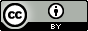# 一个 C++ 程序员眼中的 Swift（一）

1. 常量和变量

`let` 声明/定义常量，用 `var` 声明/定义变量。

``````let maxCount = 10
var currentCount = 2
``````
2. 句末分号

语句末尾不要求分号，即可有可无。

3. 类型

声明/定义常量或变量时，大部分情况下都无需指定类型。Swift 可以根据初始值或函数返回值等推断常量或变量的类型（想象一下你在 C++ 中声明一个容器的迭代器的情景）。当然，如果你不放心，也可以给 Swift 一个『提示』。

``````let name = "Swift"
let name: String = "Swift"
var red, blue, green: Int
``````
4. 常量变量名

常量变量名支持 Unicode ，你甚至可以使用 emoji ！

``````let π = 3.14159
let 你好 = "你好世界"
let 🐶🐮 = "dogcow" // 在支持 emoji 的系统上才能正常显示
``````

更有趣的是，你还可以使用 Swift 的保留字来做常量变量名，只需用 ` （数字 1 前面那个按键）把它括起来就行。

``````var `var` = "Var"
``````
5. 打印常量变量

不同于 C/C++ 中的使用格式化串作为占位符，Swift 直接把常量变量插入到要打印的串中。

``````let langName = "Swift"
println("Hello, \(langName)")
``````

这叫做『字符串插值』。在 `\()` 里还可以做更复杂的事情。

``````let π = 3.14159
var radius = 5
let message = "The circumference is \(2 * π * radius)cm"

let words = "🐶🐮"
println("The number of characters in \(words) is \(countElements(words))")
``````
6. 注释支持嵌套

这样可以方便的注释掉一段含有多行注释的代码。

7. 大一统的整型

很多 C/C++ 程序员都会郁闷的一件事是，整型 `int` 无论在 32 位平台还是在 64 位平台都只占 4 个字节。但在 Swift 里整型 `Int``UInt` 是平台相关的，即在 32 位平台上是 32 位的长度，在 64 位平台上是 64 位的长度。这看似更复杂了，但 Swift 中没有了 `short``long``long long`，也就是说整型终于大一统了。

如果体会还不深的话，那试着说出下面这几个表达式的值吧：

``````sizeof(short)
sizeof(short int)
sizeof(int)
sizeof(long)
sizeof(long int)
sizeof(long long)
sizeof(long long int)
sizeof(long double) // 这个不是整型，是来凑数的。
sizeof(void*)
sizeof(int*)
sizeof(char*)
``````
8. 基本类型其实并不是『基本类型』

Swift 中也有 `Int``Float``Double` 等这些『基本类型』。但它们其实也是『类』。

``````let maxInt = Int.max
let minInt = Int.min
``````
9. 终于有了二进制数

在 C/C++ 中我们定义二进制数的时候一般都使用十六进制 `0x` 来表示。但每次都得在脑子里转好几圈以确认是否正确。尤其是在做位运算时，十六进制真的不是那么讨人喜欢。

Swift 支持 `0b` 前缀的二进制表示。一睹为快：

``````let MaskRead = 0b0001
let MaskWrite= 0b0010
let MaskExec = 0b0100
let MaskAll  = 0b0111
``````
10. 大数中的分隔符

如果一个数很大，我们很难一眼看出它的大小。这时我们会用逗号把数字分隔开来以便识别。比如，`10000000` 写成 `1000,0000` 是不是一下就能看出是一千万？

Swift 中是这样写的：

``````let billion = 10_0000_0000
``````
11. 不再隐式类型转换

``````let i8: Int8 = Int8.max
let i16:Int16= Int16.max
let i: Int = i8 + i16 // 编译错误
let ii16: Int16 = Int16(i8) + i16 // 运行错误，因为结果已经超出 Int16
let ii: Int = Int(i8) + Int(i16) // 正确

let b = 1
if b {
// 编译错误
}

if b == 1 {
// 正确
}
``````
12. 未完待续Except where otherwise noted, content on this site is licensed under a Creative Commons Attribution 4.0 International license .